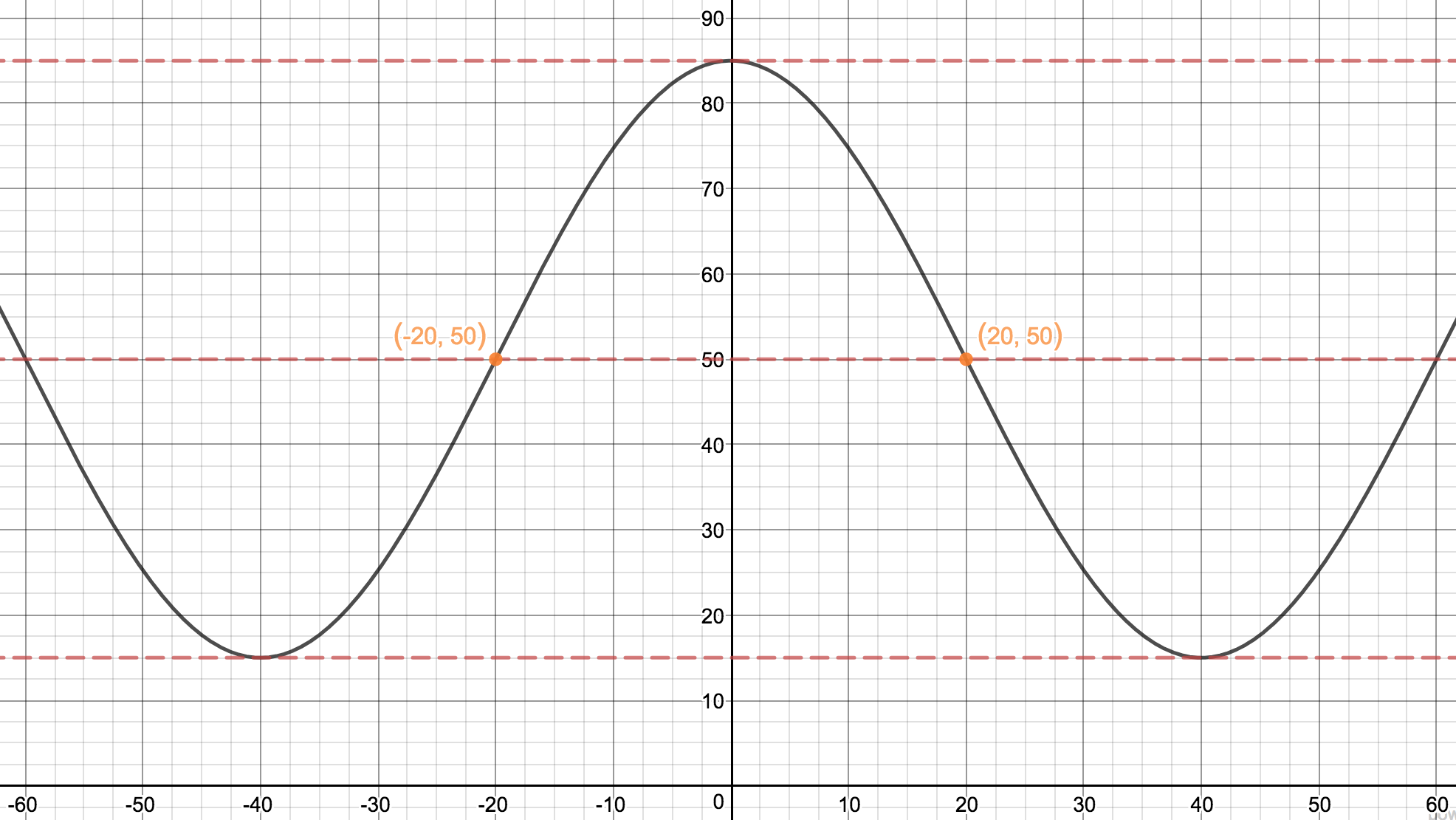### Home > PC > Chapter 7 > Lesson 7.3.3 > Problem7-127

7-127.

The graph of a transformation of $y = \cos x$ has the $y$-axis as a line of symmetry and $\left(20, 50\right)$ as a point of symmetry. The line $y = 15$ is tangent to the graph at many places. Find a possible equation.

Since $\left(20, 50\right)$ is a point of symmetry, it is a point of rotational symmetry and must be on the midline.
Since the $y$-axis is a line of symmetry, the point $\left(−20, 50\right)$ must also lie on the curve.

Since $\left(20, 50\right)$ is on the midline, the line $y = 15$ must be a lower boundary line.
Use this information to determine the amplitude and make a possible sketch.